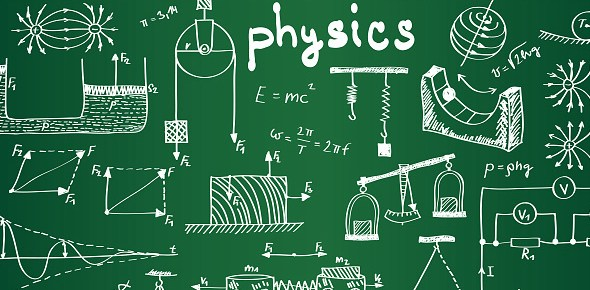# Physical Science Chapter 6 Section 1

15 Questions | Total Attempts: 64SettingsScience is a near-endless study of the universe and everything associated with it, which heavily involves the topic of physics, which deals with motion, matter, force, energy and more. What do you know about it?

• 1.
Chemical stucture determines what about a compound?
• A.

Its bond length

• B.

Its bond strength

• C.

It's weight

• D.

It's properties

• 2.
Forms when there are strong bonds between a positive sodium ion and a negative chlorine ion.
• A.

Sugar

• B.

Salt

• C.

Pepper

• D.

Quartz

• 3.
This substance forms from silicon dioxide.  The bonds that hold these atoms together are very strong
• A.

Sugar

• B.

Salt

• C.

Pepper

• D.

Quartz

• 4.
A substance formed when molecules are bonded together.  Made of carbon, hydrogen and oxygen.
• A.

Sugar

• B.

Salt

• C.

Pepper

• D.

Quartz

• 5.
Salt has the following properties.
• A.

Low melting and boiling point

• B.

High melting and boiling point

• 6.
Which of the following has a weaker bond?
• A.

Sugar

• B.

Salt

• 7.
Quartz has the following property.
• A.

Low melting and boiling point

• B.

High melting and boiling pooint

• 8.
Which of the following substances requires the most energy to break its bonds.
• A.

Sugar

• B.

Salt

• C.

Pepper

• D.

Quartz

• 9.
The force that holds atoms or ions together in a compound is known as:
• A.

Chemical strength

• B.

Chemical length

• C.

Chemical bond

• D.

Chemical structure

• 10.
Of the following models which one shows the space occupied by atoms.
• A.

Ball-stick model

• B.

Space filling model

• C.

Structural formula

• 11.
Of the following models which one uses chemical symbols to represent the atoms.
• A.

Ball-stick model

• B.

Space filling model

• C.

Structural formula

• 12.
Of the following which shows the structures of a compound.
• A.

Ball-stick model

• B.

Space filling model

• C.

Structural formula

• 13.
Of the following which represents the bond lengths and angles and the relationships between the atoms.
• A.

Ball-stick model

• B.

Space filling model

• C.

Structural formula

• 14.
Of the following which shows size.
• A.

Ball-stick model

• B.

Space filling model

• C.

Structural formula

• 15.
Which of the following is not eh best way to represent a bond because it doesn't take in to account flexibility, stretch and rotation.
• A.

Ball-stick model

• B.

Space filling model

• C.

Structural formula

Related TopicsBack to top